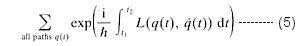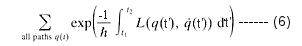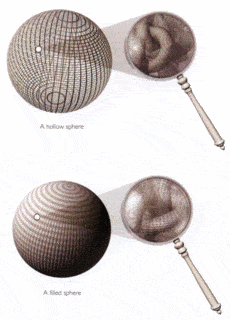# Imaginary numbers and Imaginary Time

Imaginary numbers are a lot less mysterious than they sound. They are the result from trying to take the square root of a negative number. They are called “imaginary” because they don’t exist in the normal number system, normally you can’t take the square root of a negative number because the product of two negatives is always positive. Using imaginary numbers allows physicists to plot time like is was just another dimension of space.

any product of the form ai, in which a is a real number and i is the imaginary unit defined as √(−1) .

Complex analysis
In the 18th century a far-reaching generalization of analysis was discovered, centred on the so-called imaginary number i = √(−1) . (In engineering this number is usually denoted by j.) The numbers commonly used in everyday life are known as real numbers, but in one sense this name is misleading. Numbers are abstract concepts, not objects in the physical universe. So mathematicians consider real numbers to be an abstraction on exactly the same logical level as imaginary numbers.
The name imaginary arises because squares of real numbers are always positive. In consequence, positive numbers have two distinct square roots—one positive, one negative. Zero has a single square root—namely, zero. And negative numbers have no “real” square roots at all. However, it has proved extremely fruitful and useful to enlarge the number concept to include square roots of negative numbers. The resulting objects are numbers in the sense that arithmetic and algebra can be extended to them in a simple and natural manner; they are imaginary in the sense that their relation to the physical world is less direct than that of the real numbers. Numbers formed by combining real and imaginary components, such as 2 + 3i, are said to be complex (meaning composed of several parts rather than complicated).
The first indications that complex numbers might prove useful emerged in the 16th century from the solution of certain algebraic equations by the Italian mathematicians Girolamo Cardano and Raphael Bombelli. By the 18th century, after a lengthy and controversial history, they became fully established as sensible mathematical concepts. They remained on the mathematical fringes until it was discovered that analysis, too, can be extended to the complex domain. The result was such a powerful extension of the mathematical tool kit that philosophical questions about the meaning of complex numbers became submerged amid the rush to exploit them. Soon the mathematical community had become so used to complex numbers that it became hard to recall that there had been a philosophical problem at all.

Mark44
Mentor
Physicists don't need imaginary numbers to plot time. Each point in space has three real coordinates: (x, y, z). If you also want to indicate the time relative to some specific time, you can use a fourth, real coordinate, t: (x, y, z, t).

Imaginary numbers are a lot less mysterious than they sound. They are the result from trying to take the square root of a negative number. They are called “imaginary” because they don’t exist in the normal number system, normally you can’t take the square root of a negative number because the product of two negatives is always positive. Using imaginary numbers allows physicists to plot time like is was just another dimension of space.

I don't think that they are mysterious at all, it's just a field defined differently from an ordinary Real number field

There are two very important aspects of imaginary time that are not mentioned. 1) imaginary time corresponds to equilibrium thermodynamics: if a system is held at a temperature T, one can set time= i /( k_B T ) where i is the imaginary number 2) Making this transformation converts the Schroedinger equation , which has wave solutions into a diffusion-like equation. An additional 'clue' that an imaginary time axis is relevant to real physics lies in relativistic formula for "distance" =√∆d2 - ∆t2 . If the universe is actually built on imaginary time τ ≈ i•t, then that peculiar '-' sign is there because we are [for whatever reason] using t, where squaring iτ=-t2.

Quantization of particles and fields is most elegantly prescribed by the method of path integral. The mathematical formula in term of the real time t for the probability amplitude to go from q(t1) to q(t2) is proportional to:where the sum is over all paths and L is the Lagrangian (a function of the position and velocity). The oscillating factors in this formula are very difficult to manipulate. However by substituting the real time with the imaginary time, Eq.(22a) is changed into:which become the more manageable exponentially decreasing functions. At the end of the computation, the imaginary time can be switched back to the real time.

http://www.universe-review.ca/" [Broken]

Last edited by a moderator: